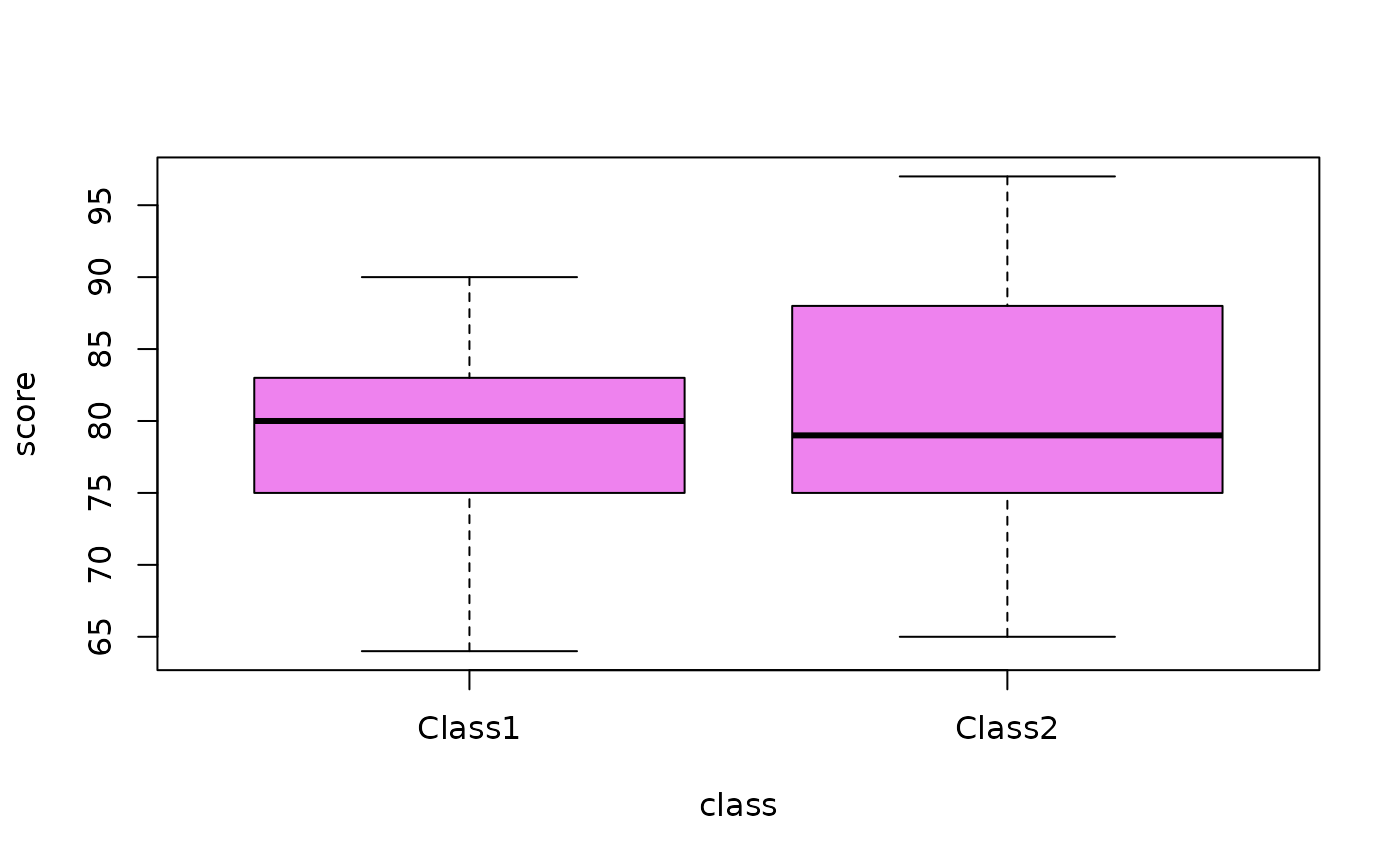Data for Exercises 1.70 and 1.87

Statisti

## Format

A data frame/tibble with 62 observations on two variables

class

character variable with values Class1 and Class2

score

test score for an introductory statistics test

## Examples


boxplot(score ~ class, data = Statisti, col = "violet")tapply(Statisti$score, Statisti$class, summary, na.rm = TRUE)
#> $Class1 #> Min. 1st Qu. Median Mean 3rd Qu. Max. #> 64.00 75.00 80.00 78.58 83.00 90.00 #> #>$Class2
#>    Min. 1st Qu.  Median    Mean 3rd Qu.    Max.    NA's
#>   65.00   75.00   79.00   80.81   88.00   97.00       4
#>
if (FALSE) {
library(dplyr)
dplyr::group_by(Statisti, class) %>%
summarize(Mean = mean(score, na.rm = TRUE),
Median = median(score, na.rm = TRUE),
SD = sd(score, na.rm = TRUE),
RS = IQR(score, na.rm = TRUE))
}